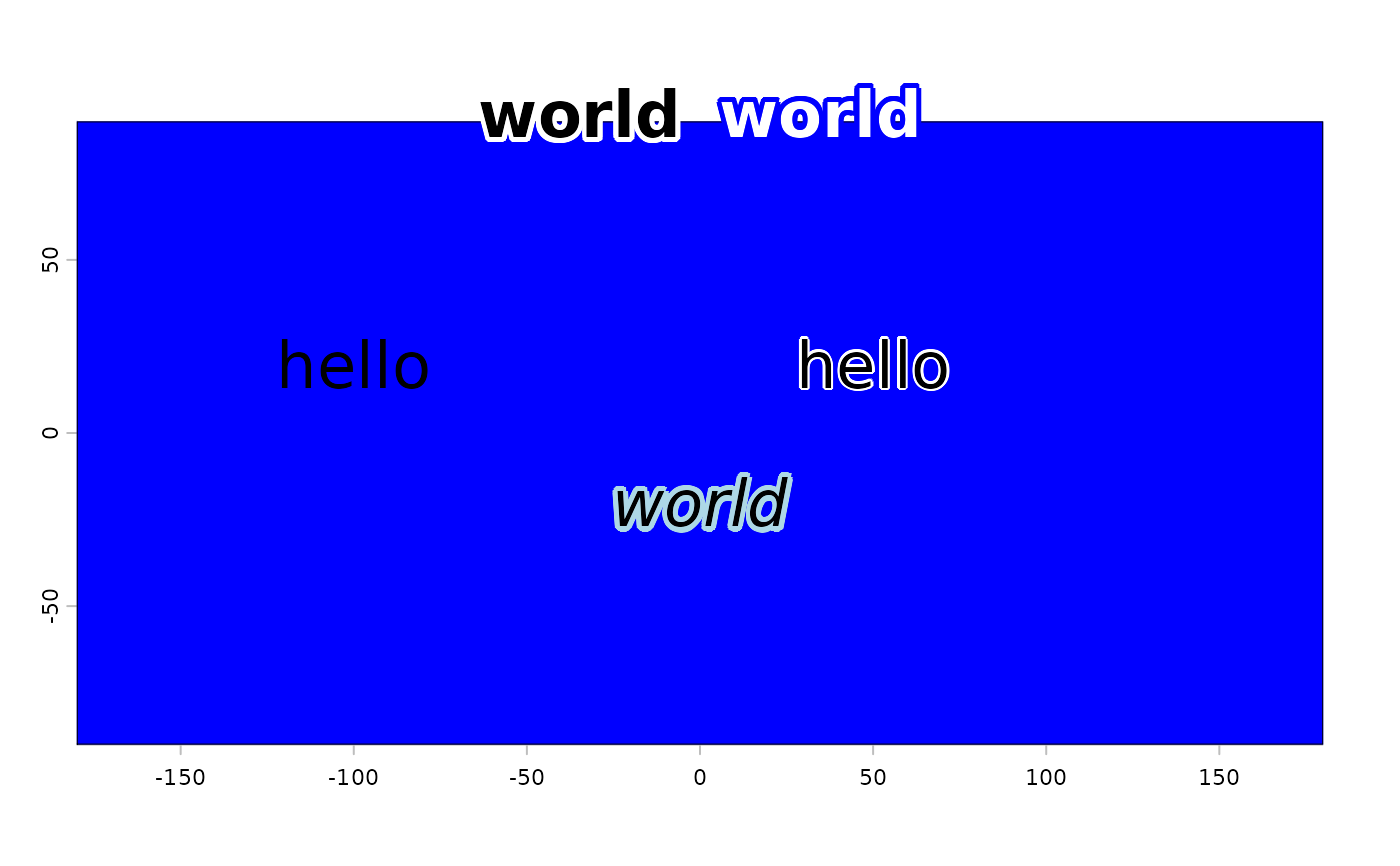Add text to a plot that has a "halo". That is, a buffer around it to enhance visibility.

halo(x, y=NULL, labels, col="black", hc="white", hw=0.1, ...)

## Arguments

x,y

numeric. coordinates where the text labels should be written

labels

character. The text to be written

col

character. The main color to be used

hc

character. The halo color

hw

numeric. The halo width

...

additional arguments to pass to text

text, plot

## Examples

r <- rast(nrows=4, ncols=4)
values(r) <- 1:ncell(r)
plot(r, col="blue", legend=FALSE)

text(-100, 20, "hello", cex=2)
halo(50, 20, "hello", cex=2)

halo(0, -20, "world", font=3, hc="light blue", cex=2, hw=.2)
halo(0, 90, "world", font=2, cex=2, hw=.2, xpd=TRUE, pos=2)
halo(0, 90, "world", col="white", font=2, hc="blue", cex=2, hw=.2, xpd=TRUE, pos=4)